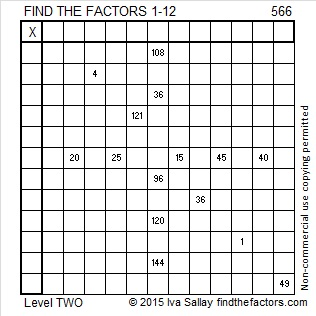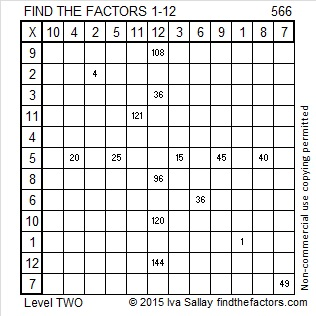# 566 and Level 2

566 is the sum of all the prime numbers from 3 to 67.Print the puzzles or type the solution on this excel file: 12 Factors 2015-07-27

—————————————————————————————————

• 566 is a composite number.
• Prime factorization: 566 = 2 x 283
• The exponents in the prime factorization are 1 and 1. Adding one to each and multiplying we get (1 + 1)(1 + 1) = 2 x 2 = 4. Therefore 566 has exactly 4 factors.
• Factors of 566: 1, 2, 283, 566
• Factor pairs: 566 = 1 x 566 or 2 x 283
• 566 has no square factors that allow its square root to be simplified. √566 ≈ 23.79075—————————————————————————————————This site uses Akismet to reduce spam. Learn how your comment data is processed.